## László Kozma :: Research

### Research interests

Data structures and algorithms, combinatorics (permutations, graphs, set systems, posets), computational geometry, machine learning.

### Publications

• Finding the saddlepoint faster than sorting
with Justin Dallant, Frederik Haagensen, Riko Jacob, Sebastian Wild, SOSA@SODA 2024.
[pdf]

• Summary: soon

• Optimization with pattern-avoiding input
with Benjamin Aram Berendsohn, Michal Opler, preprint 2023.
[pdf]

• Summary: soon

• Fast approximation of search trees on trees with centroid trees
with Benjamin Aram Berendsohn, Ishay Golinsky, Haim Kaplan, ICALP 2023.
[pdf]

• Summary: Search trees on trees (STTs) generalize binary search trees (BSTs), but computing (exact or approximate) STTs for a given distribution is much less understood than the BST case. We show that (1) centroid trees (a natural special STT) are a 2-approximation of the optimum, and (2) can be computed in O(nlogn) time (both results hold in a general, weighted case), and (3) various other centroid-related results.

• Fixed-point cycles and EFX allocations
with Benjamin Aram Berendsohn, Simona Boyadzhiyska, MFCS 2022.
[pdf]

• Summary: Suppose we label the edges of a complete bidirected graph with integers. How large can this graph be if none of the cycles has label-sum zero (modulo d). We give improved bounds for this question, also simplifying earlier proofs. We also look at generalizations where the labels are permutations or arbitrary functions [d]->[d]. The more general question has connections with a fair allocation problem.
Follow-up: Similar results and improvements are shown in independent work [here] and [here].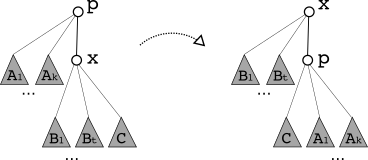• Splay trees on trees
with Benjamin Aram Berendsohn, SODA 2022.
[pdf]

• Summary: Search trees on trees (STTs) generalize binary search trees (BSTs): whereas BSTs search over a linear search space, SSTs search over a tree. We show the following: (1) a poly-time algorithm to find an (1+eps)-approximation of the optimal STT for a given search distribution, (2) linear rotation-distance for a broad class of STTs, (3) a generalization of Splay trees to STTs, (4) static optimality of this generalized Splay, asymptotically matching the optimum tree (without knowing the search distribution).

• Analysis of smooth heaps and slim heaps
with Maria Hartmann, Corwin Sinnamon, Robert E. Tarjan, ICALP 2021.
[pdf] [smooth-heap.c] [slim-heap.c] [slides]

• Summary: We study the smooth heap (introduced in STOC 2018 paper, see below), and a closely related (simpler) heap we call the slim heap, giving a self-contained analysis of both data structures (previously smooth heaps were analyzed in a restricted setting only). We describe and analyse different implementations of operations. The results (for a certain variant) match the best bounds known for self-adjusting heaps. We also test smooth and slim heaps experimentally, observing that they compare favorably with pairing heaps.
Follow-up: In SODA 2023 [ref], Sinnamon and Tarjan prove a related stronger result, showing that the bounds for smooth and slim heaps hold even for the simplest and most natural implementation, matching a known lower bound for self-adjusting heaps. This makes smooth/slim heaps the first (and so far the only known) optimal self-adjusting heaps. See Sinnamon's [thesis] for the full set of results.

• Group testing with geometric ranges
with Benjamin Aram Berendsohn, ISIT 2022.
[pdf] [compact-pdf]

• Summary: Given m objects (points in d-dimensional space), how many tests (d-dimensional axis-parallel boxes) are needed to identify t defective objects? A test reports whether at least one of the objects in it is defective, and all tests are run in parallel. We introduce this geometric version of the classical group testing problem and give upper and lower bounds for various values of t and d that improve on the trivial bounds.

• Exact exponential algorithms for two poset problems
SWAT 2020.
[pdf] [talk-notes]

• Summary: Exact exponential-time algorithms are given for the following problems: counting linear extensions when the input poset is two-dimensional, and the jump number problem in arbitrary posets (both running times: ~1.82^n).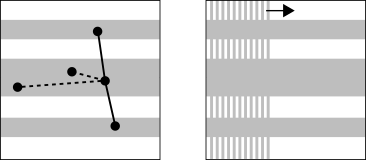• Finding and counting permutations via CSPs
with Benjamin Aram Berendsohn, Dániel Marx, IPEC 2019.
(Journal version: Algorithmica, Vol 83(8), 2021, by invitation.)

• Summary: The permutation pattern problem asks whether a given permutation of length n contains a given pattern of length k. We give two new algorithms for the problem: one with running time n^{0.25k+o(k)}, and one with running time O(1.6181^n) or n^{0.5k+o(k)}. The results improve the earlier best bounds for the problem for patterns of size k≈log(n) or larger and reduce the problem to constraint-satisfaction. Our second algorithm uses polynomial space and is simpler than previous approaches for the problem. We include hardness results and improved bounds for special cases, and the counting version of the problem is also studied.
Berendsohn's thesis includes refinements of the hardness results with more details. The paper supersedes an earlier report [preprint].
Follow-up: The O(1.618^n) approach is improved to O(1.415^n) through a simple (but clever) modification by Gawrychowski and Rzepecki [preprint]. Some refined hardness results appear [here].

• Hamiltonicity below Dirac's condition
with Bart Jansen, Jesper Nederlof, WG 2019.
[pdf] [slides]

• Summary: Dirac's theorem (1952) is a classical result of graph theory, stating that an n-vertex graph is Hamiltonian if every vertex has degree at least n/2. We show that there are efficient algorithms to determine the Hamiltonicity of a graph, when the degree-requirements of Dirac's theorem are relaxed: we either let the minimum degree be n/2-k, or we let some k vertices have arbitrary degree.
Follow-up: In SODA 2022, Fomin, Golovach, Sagunov, and Simonov [preprint] provide a generalization, confirming the main conjecture of our paper.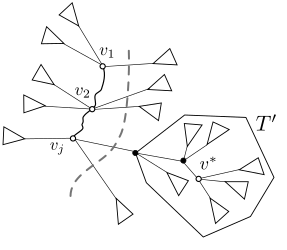• Time- and space-optimal algorithm for the many-visits TSP
with André Berger, Matthias Mnich, Roland Vincze, SODA 2019.
(Journal version: ACM TALG, Vol 16(3), 2020.)

• Summary: In the many-visits TSP, a salesperson must visit n cities, each of them precisely a prescribed number of times (in the cheapest possible way). We give a polynomial-space algorithm for this problem, with running time that depends single-exponentially on n. This improves on the previous best algorithm by Cosmadakis and Papadimitriou from 1984, that has superexponential time- and space-complexity.
Follow-up: The running time of our algorithms is of the form c^n with c=16 (when polynomial space is used) and c=5 (with exponential space). In ESA 2020, Kowalik, Li, Nadara, Smulewicz, and Wahlström [preprint] improve the first to c≈7.88 and refine the analysis of the second to c=4.

• Selection from heaps, row-sorted matrices and X + Y using soft heaps
with Haim Kaplan, Or Zamir, Uri Zwick, SOSA@SODA 2019.
[pdf] [slides -- Uri Zwick]

• Summary: Using soft heaps, we obtain simpler optimal algorithms for selecting the k-th smallest item from heap-ordered trees, from sets of sorted lists, and from sets of pairwise sums (X + Y), matching, and in some ways extending classical results of Frederickson (1993) and Frederickson and Johnson (1982).

• Improved bounds for multipass pairing heaps and path-balanced binary search trees
with Dani Dorfman, Haim Kaplan, Seth Pettie, Uri Zwick, ESA 2018.
[pdf]

• Summary: We improve the analysis of a classical pairing heap variant (Fredman, Sedgewick, Sleator, Tarjan, 1985) and a classical self-adjusting binary search tree heuristic (Sleator, 198x), reducing the gap to the information-theoretic lower bound from roughly (loglog n) to less than (loglog...log n), where log(.) can be iterated any constant number of times.
Follow-up: For multipass pairing heaps further improvements are obtained in SODA 2023 by Sinnamon and Tarjan [link].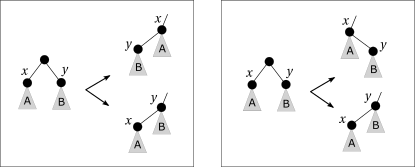• Smooth heaps and a dual view of self-adjusting data structures
with Thatchaphol Saranurak, STOC 2018, HALG 2019.
(Journal version: SICOMP, Vol 49(5), 2020, by invitation.)
[pdf] [slides] [poster -- Thatchaphol] [journal link] [smooth-heap.c] [slim-heap.c]

• Summary: We describe a new connection ("duality") betweden self-adjusting binary search trees (BSTs) and heaps. This allows us to transfer results between the two settings, obtaining: (1) a broad class of "stable" heap algorithms, (2) instance-specific lower and upper bounds for stable heaps, (3) a new heap data structure called "smooth heap", which we show to be the heap-counterpart of a BST that is conjectured to be "instance-optimal", (4) new "offline" BST algorithms.
Follow-up: In further work more general analyses of smooth heaps and their variants have been given (see above).

• Multi-finger binary search trees
with Parinya Chalermsook, Mayank Goswami, Kurt Mehlhorn, Thatchaphol Saranurak, ISAAC 2018.
[pdf] [slides]

• Summary: We show that Binary Search Trees (BSTs) with multiple fingers can be efficiently simulated by standard (one-finger) BSTs with rotations. The results connect two prominent online problems: dynamic BSTs and k-server. As an application, we show that BSTs can take advantage of certain regularities in the input, for instance, when accessing items close to some recently accessed item.
Related: The paper builds upon (and partially supersedes) the technical report The landscape of bounds for binary search trees. [pdf]
Some results are found only in the report: a survey of BST properties; new connections/separations between BST properties; a new, more intuitive analysis of Splay trees; a technique for composing ("interleaving") sequences and combining BST properties; an observation about the Move-to-root heuristic.

• Pairing heaps: the forward variant
with Dani Dorfman, Haim Kaplan, Uri Zwick, MFCS 2018.
[pdf] [slides]

• Summary: We give a new analysis of a classical pairing heap variant (Fredman, Sedgewick, Sleator, Tarjan, 1985), improving the bound on the cost of operations from O(n^0.5) to better than O(n^eps) for any constant eps>0. The main novelty is a potential function that captures rank-differences between nodes.• Maximum Scatter TSP in Doubling Metrics
with Tobias Mömke, SODA 2017.
[pdf] [slides, extra slides]

Summary: We give a polynomial time (1+eps)-approximation for euclidean (and more general) cases of the Traveling-Salesman variant in which no hop may be too short, improving on the earlier best ratio of 2.
Related: Supersedes preliminary paper presented at EuroCG 2016. [pdf]

• Binary search trees, rectangles and patterns, PhD thesis, Saarland University, 2016.
[pdf] [slides]

• Summary: Contains results related to binary search trees and the dynamic optimality conjecture from three papers, expanded with a survey of the problem, and some new observations.

• Binary search trees and rectangulations
with Thatchaphol Saranurak, Technical report (work-in-progress), 2016.
[pdf]

• Summary: Serving a sequence of searches in a binary search tree with rotations is shown to be equivalent with the previously studied problem of finding a sequence of flips between two rectangulations. Connections of both problems to Manhattan networks are also explored.
Related: The core idea of heap/BST duality appears in this paper, developed further in our STOC 2018 smooth heap paper (above). The material also appears (with small updates) as Chapter 5 of my thesis.

• Hitting Set for hypergraphs of low VC-dimension
with Karl Bringmann, Shay Moran, N.S. Narayanaswamy, ESA 2016.
[pdf]

• Summary: The Hitting Set problem remains hard (in the parameterized sense), even in set systems of very small VC-dimension. If we restrict the structure even more, then Hitting Set is solvable in polynomial time. Our easy class is a generalization of Edge Cover.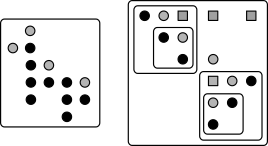• Pattern-avoiding access in binary search trees
with Parinya Chalermsook, Mayank Goswami, Kurt Mehlhorn, Thatchaphol Saranurak, FOCS 2015, HALG 2016.
[pdf] [slides I -- Thatchaphol] [slides II]

• Summary: Searches in binary search trees (BSTs) take almost constant (amortized) time if the search sequence avoids an arbitrary fixed pattern. Moreover, this is attained by Greedy, a general-purpose BST algorithm that is conjectured optimal (for every sequence). Pattern avoiding sequences generalize some well-studied earlier examples, which can be seen as avoiding some particular pattern. Many of the arguments rely on results from forbidden submatrix theory. Part of the material appears (with some updates) as Chapter 4 of my thesis.

• Greedy Is an Almost Optimal Deque
with Parinya Chalermsook, Mayank Goswami, Kurt Mehlhorn, Thatchaphol Saranurak, WADS 2015.
[pdf] [slides -- Thatchaphol]

• Summary: We extend the "geometry of BST" model of Demaine et al. to handle insert and delete operations, and we show that the Greedy algorithm is almost optimal on deque sequences (where insert and delete happens only at the minimum and maximum). The results give circumstantial evidence that Greedy may be instance-optimal.• Self-Adjusting Binary Search Trees: What Makes Them Tick?
with Parinya Chalermsook, Mayank Goswami, Kurt Mehlhorn, Thatchaphol Saranurak, ESA 2015.
[pdf] [slides]

• Summary: We give combinatorial conditions that guarantee the efficiency of algorithms for re-arranging binary search trees. This unifies the analysis of several known heuristics, and leads to some new ones that are based on depth-reduction. We also characterize all tree re-arrangement strategies that are local.
Related: The paper supersedes an earlier draft note [pdf] which may nonetheless contain additional intuition. Part of the material appears (with some updates) as Chapter 3 of my thesis.

• Streaming Algorithms for Partitioning Integer Sequences
[pdf]

• Summary: We study the problem of partitioning a stream of integers into blocks with roughly equal total value, in one pass. We look at the trade-off between approximation-ratio and amount of memory used.

• Shattering, Graph Orientations, and Connectivity
with Shay Moran, Electronic Journal of Combinatorics, Vol 20(3), 2013.
[pdf] [slides]

• Summary: We interpret results for set systems, shattering, and VC-dimension, in the context of graph orientations. We thus obtain a number of inequalities (some known, some new) involving distances, flows, connectivity, forbidden subgraphs, etc., in graphs. A warm-up example: the number of orientations of a graph G in which there is an s-to-t directed path equals the number of spanning subgraphs of G in which s and t are connected, for every G, s, t.

• Privacy by Fake Data: A Geometric Approach
with Victor Alvarez, Erin Chambers, CCCG 2013.
[pdf]

• Summary: Given n points in d dimensions, add as few extra points as possible, so that no point can be isolated within a unit box. The problem arises in the context of data privacy. We give approximation and hardness results.• Minimum Average Distance Triangulations
ESA 2012.
[pdf] [slides] [poster]

• Summary: How to connect n points in the plane with a non-crossing network, so that the average distance between a pair of points is as small as possible. In general, the problem is shown to be hard, in the unit-weight case a non-trivial polynomial-time algorithm is found, and the metric/euclidean case is left open.

• Binary Principal Component Analysis in the Netflix Collaborative Filtering Task
with Alexander Ilin, Tapani Raiko, MLSP 2009.
[pdf] [slides]

• Summary: We propose a PCA-like factorization algorithm for binary matrices with missing values, that scales well to very high dimensional and very sparse data. Together with a binarization method, the algorithm directly reconstructs integer entries within a small range, it is thus well-suited for predicting ratings in collaborative filtering.